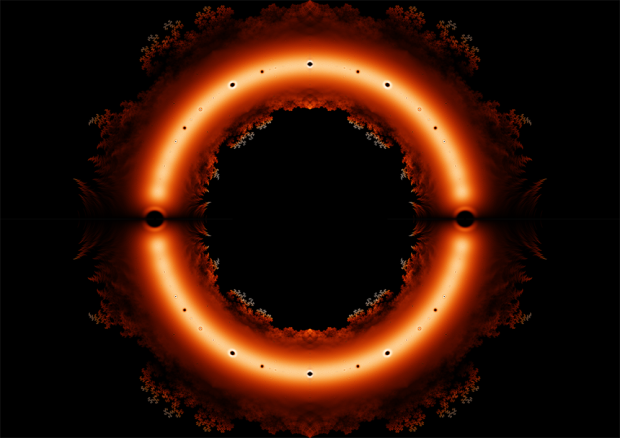## 绘制整系数多项式复数根的分布• 如何求解多项式的根
• 图上的颜色按照什么绘制

## C++的直接初始化与复制初始化

C++中的直接初始化指的是直接调用类的构造函数进行初始化，例如

string a; //调用默认构造函数
string a("hello"); //调用参数为const char *类型的构造函数
string b(a); //调用拷贝构造函数


string a="hello";
string b=a;


## 为什么C++直接输出21000可以精确到个位

#include<iostream>
#include<cmath>

using namespace std;

int main(){
int x;
cin>>x;
cout.precision(0);
cout<<fixed<<pow(2,x);
return 0;
}


## C/C++中的const可信吗？

C和C++中有很多函数的参数类型使用了const这个关键字。例如C语言string.h头文件中的strcpy字符串复制函数，它的原型是

char * strcpy (char * Dest, const char * Source)


void qsort (void * Base, size_t NumOfElements, size_t SizeOfElements, int (*PtFuncCompare)(const void *, const void *))


## 【π Day】一段很短的计算圆周率的代码

#include<stdio.h>
int a=10000,b=0,c=28000,d,e=0,f,g;
main(){
for(;b-c;)f[b++]=a/5;
for(;d=0,g=c*2;c-=14,printf("%04d",e+d/a),e=d%a)
for(b=c;d+=f[b]*a,f[b]=d%--g,d/=g--,--b;d*=b);
}


## C语言中的gets()与fgets()

C语言中gets(char*);可以从标准输入中读入一行字符，存入字符串；而用fgets(char*,int,FILE*)可以从文件读入一行字符，其中的整型参数指的是缓冲区大小。

## scanf函数中的扫描集

ANSI C语言标准向scanf函数增加了一种新特性，叫做扫描集。利用此特性可以解决一些处理文本时的棘手问题。

char a,b;
scanf("%s,%s",a,b);


Alice,Bob

## 从图片看出伪随机数的周期性